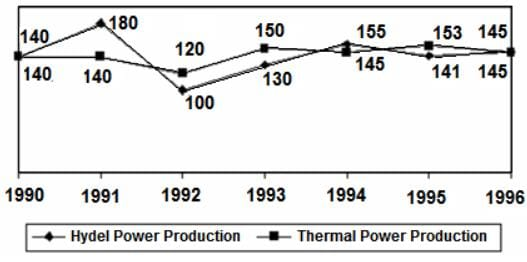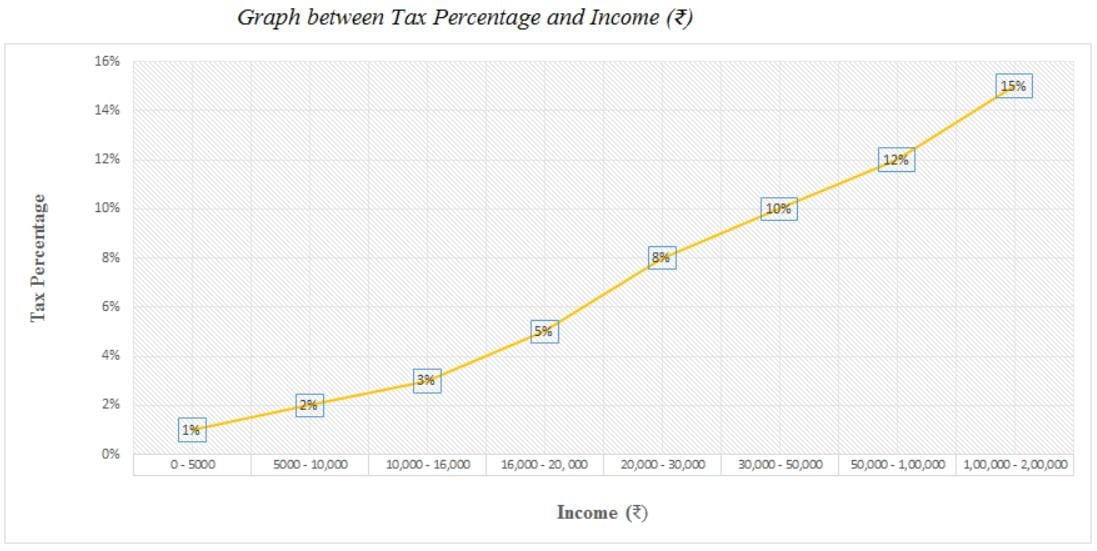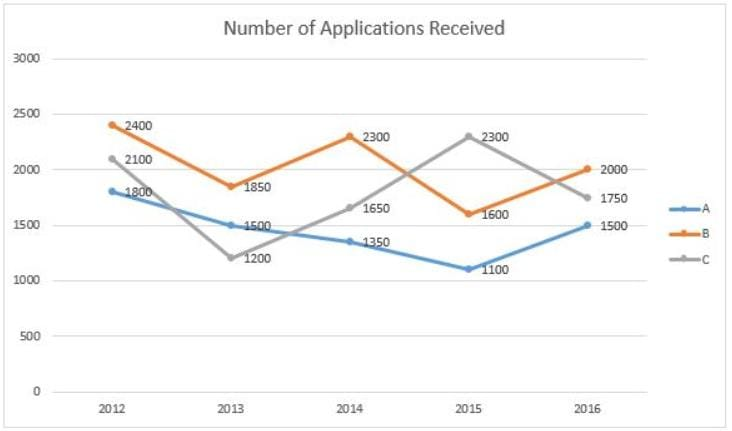CAT  >  Practice Questions Level 1: Line Graph

# Practice Questions Level 1: Line Graph - Notes | Study Level-wise Practice Questions for CAT Preparation - CAT

 1 Crore+ students have signed up on EduRev. Have you?

This EduRev document offers 10 Multiple Choice Questions (MCQs) from the topic line Graph (Level - 1). These questions are of Level - 1 difficulty and will assist you in the preparation of CAT & other MBA exams. You can practice/attempt these CAT Multiple Choice Questions (MCQs) and check the explanations for a better understanding of the topic.

Question for Practice Questions Level 1: Line Graph
Try yourself:Directions: Study the following graph and answer the question that follows.

POWER PRODUCTION OF A COMPANY (IN MILLION WATTS)Question for Practice Questions Level 1: Line Graph
Try yourself:Directions: Study the following graph and answer the question that follows.

POWER PRODUCTION OF A COMPANY (IN MILLION WATTS)Q. In which year is the total power production (thermal + hydel) the greatest?

Question for Practice Questions Level 1: Line Graph
Try yourself:Directions: Study the following graph and answer the question that follows.

POWER PRODUCTION OF A COMPANY (IN MILLION WATTS)Q. What is the average thermal power production for the period 1990-1996?

Question for Practice Questions Level 1: Line Graph
Try yourself:Directions: Study the following graph carefully and answer the question given below.Q. If the population of a city is 35,000 and the average income of a person in that city is Rs. 25,000 per annum, then what is the total amount of tax paid by all the persons of that city?

Question for Practice Questions Level 1: Line Graph
Try yourself:Directions: Study the following graph carefully and answer the question given below.Q. What is the total amount of tax on an income of Rs. 9000 and an income of Rs. 2000?

Question for Practice Questions Level 1: Line Graph
Try yourself:Directions: Study the following graph carefully and answer the question given below.Q. What is the ratio of the tax on an income of Rs. 15,550 to that on an income of Rs. 16,550?

Question for Practice Questions Level 1: Line Graph
Try yourself:Directions: Refer to the graph and answer the following question.

The line graph below gives information about the number of applications received by different colleges A, B and C for admission to their MBA programme from 2012 to 2016.Q. What is the ratio of the number of applications received by colleges A, B and C in 2013 to that in 2016?

Question for Practice Questions Level 1: Line Graph
Try yourself:Directions: Refer to the graph and answer the following question.

The line graph below gives information about the number of applications received by different colleges A, B and C for admission to their MBA programme from 2012 to 2016.Q.  What is the difference between the number of applications received by college C during 2013-2016 and that received by college A during 2012-2015?

Question for Practice Questions Level 1: Line Graph
Try yourself:Directions: Refer to the graph and answer the following question.

The line graph below gives information about the number of applications received by different colleges A, B and C for admission to their MBA programme from 2012 to 2016.Q. Out of total applications received by colleges A, B and C in 2014, 40% were shortlisted by college A, 35% were shortlisted by college B and 60% were shortlisted by college C for further rounds. How many applicants were shortlisted by colleges A, B and C together in 2014?

Question for Practice Questions Level 1: Line Graph
Try yourself:Directions: Refer to the graph and answer the following question.

The line graph below gives information about the number of applications received by different colleges A, B and C for admission to their MBA programme from 2012 to 2016.Q. Total applications received by college A in years 2012, 2013 and 2014 are what percent (approximately) of those received by college B in years 2014, 2015 and 2016?

The document Practice Questions Level 1: Line Graph - Notes | Study Level-wise Practice Questions for CAT Preparation - CAT is a part of the CAT Course Level-wise Practice Questions for CAT Preparation.
All you need of CAT at this link: CAT

## Level-wise Practice Questions for CAT Preparation

277 docs
 Use Code STAYHOME200 and get INR 200 additional OFF

## Level-wise Practice Questions for CAT Preparation

277 docs

### How to Prepare for CAT

Read our guide to prepare for CAT which is created by Toppers & the best Teachers

Track your progress, build streaks, highlight & save important lessons and more!

,

,

,

,

,

,

,

,

,

,

,

,

,

,

,

,

,

,

,

,

,

;